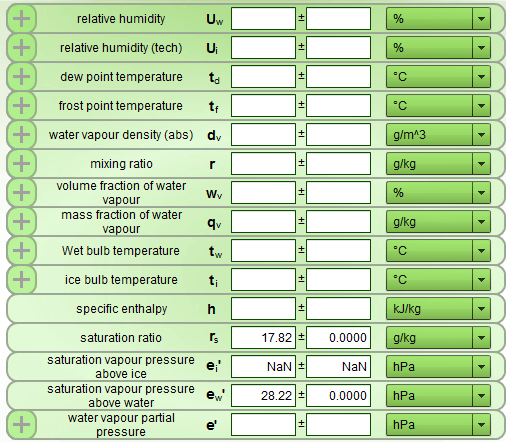# Humidity calculator

The humidity calculator is used for the rapid conversion of humidity measurements. Uniquely, the humidity calculator also includes measurement uncertainties in the calculation. This is helpful for obtaining realistic and reliable overall uncertainties based on the specification of the measuring device.The simple and intuitive operation of the humidity calculator facilitates the work. The resulting humidity measurements are displayed immediately, subject to changing ambient conditions.

Simulations and calculations can be copied from the humidity calculator to Excel for further processing and saved in PDF format for documentation purposes.

The humidity calculator can be used online. This does not require any program to be installed on your PC.

Explanation of humidity calculator measurements

Water vapour pressure

Pressure of the gaseous phase of water in a given volume in pure phase or of the water vapour partial pressure in an ideal gas or gas mixture, respectively.
Symbol: e
Unit: hPa

Saturation vapour pressure above water

Vapour pressure in a real gas or gas mixture under saturation conditions above water.
Symbol: ew
Unit: hPa

Saturation vapour pressure above ice

Vapour pressure in a real gas or gas mixture under saturation conditions above ice.
Symbol: ei
Unit: hPa

Relative humidity

Percentage of water vapour partial pressure to saturation vapour pressure under saturation conditions above water and at equal total pressure and temperature.
Symbol: Uw
Unit: %

Relative humidity (technical definition)

For temperatures t < 0 °C, the relative humidity is related to the saturation vapour pressure above ice ei. Percentage of water vapour partial pressure to saturation vapour pressure under saturation conditions above ice and at equal total pressure and temperature.
Symbol: Ui
Unit: %

Dewpoint temperature

The temperature at which the prevailing water vapour partial pressure is equal to the saturation vapour pressure and at which condensation starts.
Symbol: td
Unit: °C

Frostpoint temperature

The temperature at which the prevailing water vapour partial pressure is equal to the saturation vapour pressure and at which ice begins to form.
Symbol: tf
Unit: °C

Water vapour density (abs); absolute humidity

Ratio of the mass of water vapour to the volume in which the humid gas is contained. Zmix is the compression factor of the mixed gas system.
Symbol: dv
Unit: kg/m3

Mixing ratio

Ratio of mass of water vapour to mass of dry gas.
Symbol: r
Unit: g/kg

Volume fraction of water vapour

Ratio of volume fraction of water vapour to total volume of humid gas.
Symbol: wv
Unit: %

Mass fraction of water vapour

Ratio of mass of water vapour to mass of humid gas.
Symbol: qv
Unit: g/kg

Wet bulb temperature, ice bulb temperature

Temperature resulting at the interface between a wetted or iced surface and a flowing gas.
Symbol: tw, ti
Unit: °C

Specific enthalpy

Parameter of state of the humid gas, composed of the specific enthalpies of the components of the mixture and related to the mass fraction of the dry gas. cpa is the specific heat capacity of the dry gas, cpv is the specific heat capacity of water vapour, each at constant pressure, and lw is the specific latent heat (evaporation heat) of water.
Symbol: h
Unit: J/kg

Mixing ratio at saturation

Ratio of mass of water vapour at saturation to mass of dry gas.
Symbol: rw
Unit: g/kg

1.